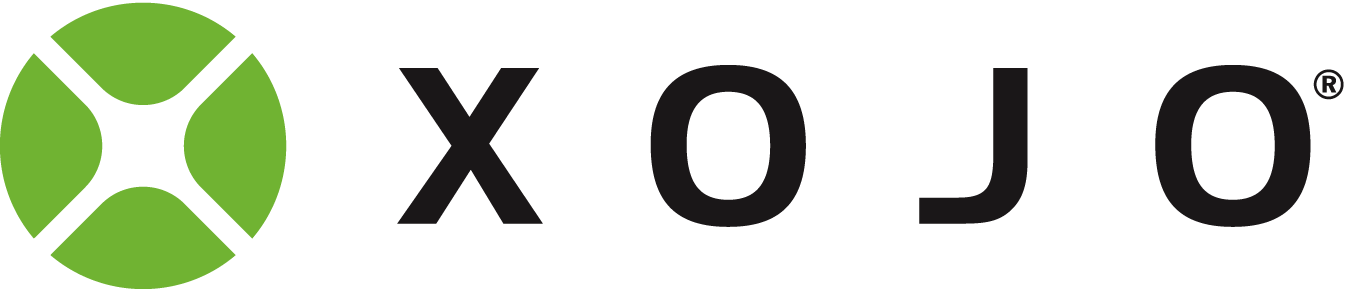# Odd value of color.value

Xojo 2018 R3 on a Mac

For a color C which is &c0000FE00 in the debugger, I am getting a
c.value of 0.99
This seems way off to me… near white

Anyone have an idea why?

Hi @Jeff_Tullin,

Maybe you already know this and I don’t really understand the problem you’re facing…

In the HSV color model, “V” (Value) stands for the amount of brightness, while “H” (Hue) stands for the tint and “S” for the color saturation.

So if you only read for the “Value” component of the model, getting 0.99 seems right to me (the possible range is from 0 to 1).

That I thought I understood. ‘how dark is it’?

But this color is not ‘almost as bright as a color could be’ (ie nearly 1)
It’s as if it is only considering the blue component… adding red or green couldn’t increase that.

What I am really looking for is ‘how dark is this color’, in order to decide a threshold beyond which text drawn on top should be black or white.

I have been using if c.Value < 0.6 then and it doesnt seem accurate enough now

``````Dim col As color = someColorValue

Dim luminance As Double = (0.2126*col.red + 0.7152*col.green + 0.0722*col.Blue)/255.0

if luminance < 0.5 then

//Do something
else
//Do something else

end if
``````
2 Likes

Maybe you need HSL rather than HSV.

``````Sub RGB2HSL(pRGBColour As Color, ByRef pH As Double, ByRef pS As Double, ByRef pL As Double)
Dim r, g, b As Double
Dim h, s, l As Double
Dim cMax, cMin As Double
Dim delta As Double

h = 0.0
s = 0.0
l = 0.0

r = pRGBColour.Red / 255
g = pRGBColour.Green / 255
b = pRGBColour.Blue / 255

cMax = Max(r, Max(g, b))
cMin = Min(r, Min(g, b))

l = (cMax + cMin) / 2.0

If cMax = cMin Then
s = 0.0
h = 0.0
Else
If l < 0.5 Then
s = (cMax - cMin) / (cMax + cMin)
Else
s = (cMax - cMin) / (2.0 - cMax - cMin)
End If

delta = cMax - cMin

If r = cMax Then
h = (g - b) / delta
ElseIf g = cMax Then
h = 2.0 + (b - r) / delta
Else
h = 4.0 + (r - g) / delta
End If

h = h / 6.0

If h < 0.0 Then
h = h + 1
End If
End If

h = h * 255.0
s = s * 255.0
l = l * 255.0

pH = h
pS = s
pL = l
End Sub``````

From what I see, although .VALUE is described as brightness, it is in fact the brightness of the brightest R,G, or B
Luminescence does seem closer to what I need.

Forum for Xojo Programming Language and IDE. Copyright © 2021 Xojo, Inc.